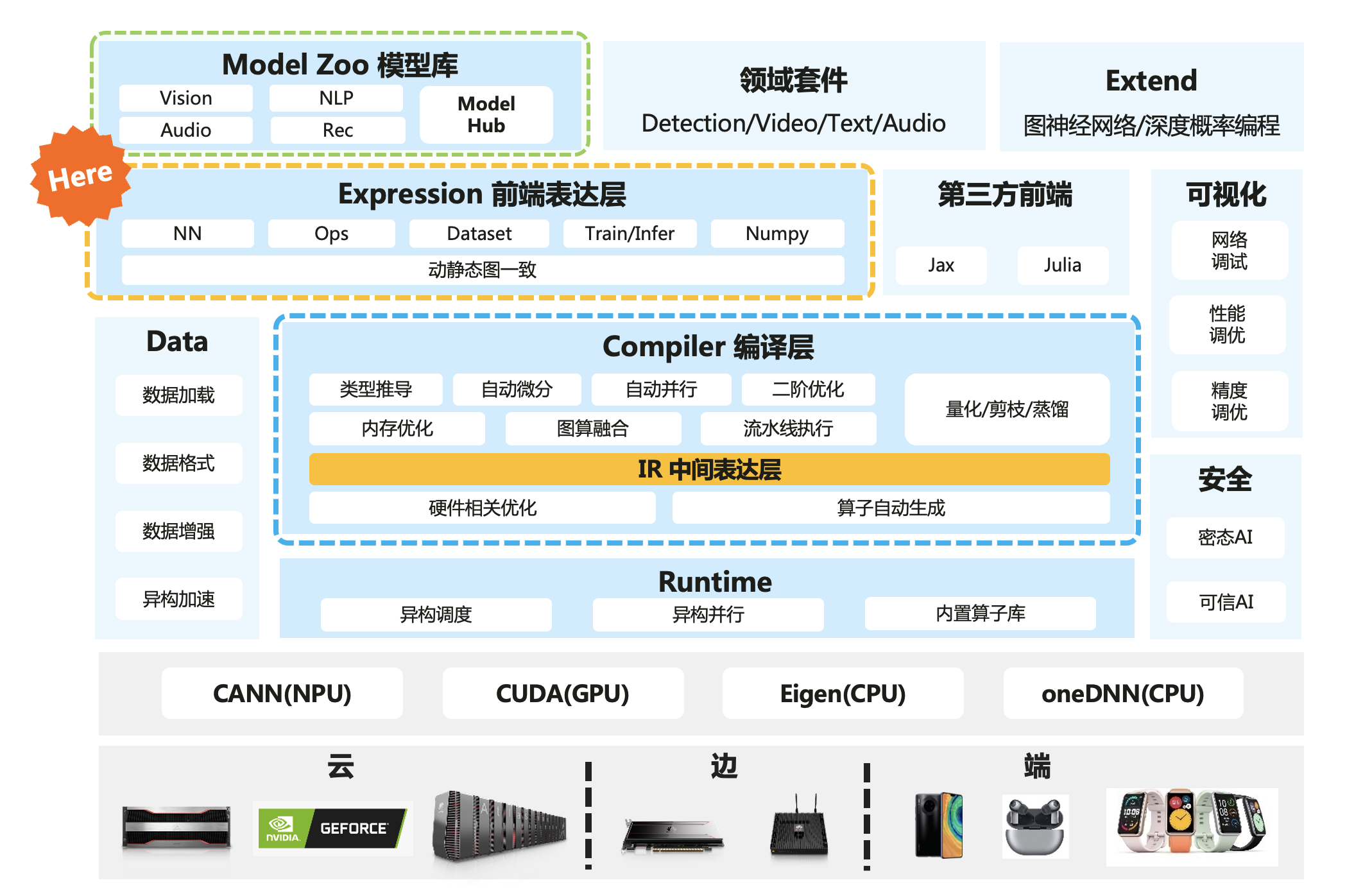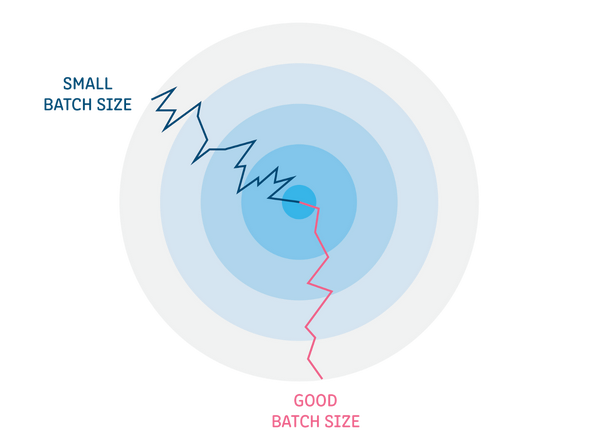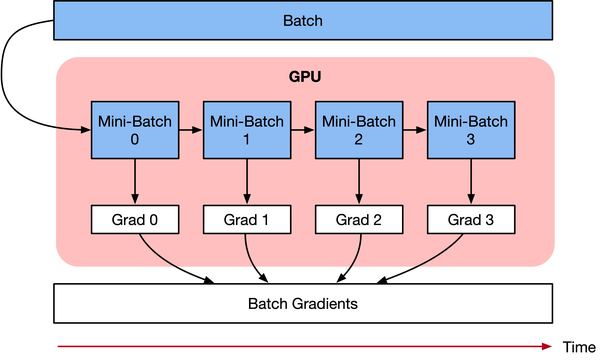# AI系统——梯度累积算法

01/08 21:36

• 明天博士论文要答辩了，只有一张12G二手卡，今晚通宵要搞定10个模型实验

• 挖槽，突然想出一个T9开天霹雳模型，加载不进去我那张12G的二手卡，感觉要错过今年上台Best Paper领奖## Batch size的作用

> 不同的神经网络和不同的数据集可能有不同的最佳Batch size大小。### Batch size对内存的影响

• 模型参数：网络模型需要用到的权重参数和偏差。

• 优化器变量：优化器算法需要的变量，例如动量momentum。

• 中间计算变量：网络模型计算产生的中间值，这些值临时存储在AI加速芯片的内存中，例如，每层激活的输出。

• 工作区Workspace：AI加速芯片的内核实现是需要用到的局部变量，其产生的临时内存，例如算子D=A+B/C中B/C计算时产生的局部变量。

### 使用大Batch size的方法

1）数据并行：使用多个AI加速芯片并行训练所有Mini-Batch，每份数据都在单个AI加速芯片上。累积所有Mini-Batch的梯度，结果用于在每个Epoch结束时求和更新网络参数。

2）梯度累积：按顺序执行Mini-Batch，同时对梯度进行累积，累积的结果在最后一个Mini-Batch计算后求平均更新模型变量。

## 梯度累积原理

$Loss(\theta)=\frac{1}{2}\left(h(x^{k})-y^{k}\right)^{2}$

$\theta{i}=\theta_{i-1}-lr * grad_{i}$

$accumulated=\sum_{i=0}^{N} grad_{i}$

$\theta{i}=\theta_{i-1}-lr * \sum_{i=0}^{N} grad_{i}$> 学习率 learning rate：一定条件下，Batch size越大训练效果越好，梯度累积则模拟了batch size增大的效果，如果accumulation steps为4，则Batch size增大了4倍，根据ZOMI的经验，使用梯度累积的时候需要把学习率适当放大。 归一化 Batch Norm：accumulation steps为4时进行Batch size模拟放大效果，和真实Batch size相比，数据的分布其实并不完全相同，4倍Batch size的BN计算出来的均值和方差与实际数据均值和方差不太相同，因此有些实现中会使用Group Norm来代替Batch Norm。

## 梯度累积实现

for i, (images, labels) in enumerate(train_data):
# 1. forwared 前向计算
outputs = model(images)
loss = criterion(outputs, labels)

# 2. backward 反向传播计算梯度
loss.backward()
optimizer.step()

• model(images) 输入图像和标签,前向计算。
• criterion(outputs, labels) 通过前向计算得到预测值，计算损失函数。
• ptimizer.zero_grad() 清空历史的梯度信息。
• loss.backward() 进行反向传播，计算当前batch的梯度。
• optimizer.step() 根据反向传播得到的梯度，更新网络参数。

# 梯度累加参数
accumulation_steps = 4

for i, (images, labels) in enumerate(train_data):
# 1. forwared 前向计算
outputs = model(imgaes)
loss = criterion(outputs, labels)

# 2.1 loss regularization loss正则化
loss += loss / accumulation_steps

# 2.2 backward propagation 反向传播计算梯度
loss.backward()

# 3. update parameters of net
if ((i+1) % accumulation)==0:
# optimizer the net
optimizer.step()

• model(images) 输入图像和标签,前向计算。
• criterion(outputs, labels) 通过前向计算得到预测值，计算损失函数。
• loss / accumulation_steps loss每次更新，因此每次除以steps累积到原梯度上。
• loss.backward() 进行反向传播，计算当前batch的梯度。
• 多次循环伪代码步骤1-2，不清空梯度，使梯度累加在历史梯度上。
• optimizer.step() 梯度累加一定次数后，根据所累积的梯度更新网络参数。
• optimizer.zero_grad() 清空历史梯度，为下一次梯度累加做准备。

## 参考文献### 作者的其它热门文章

0
0 收藏

0 评论
0 收藏
0